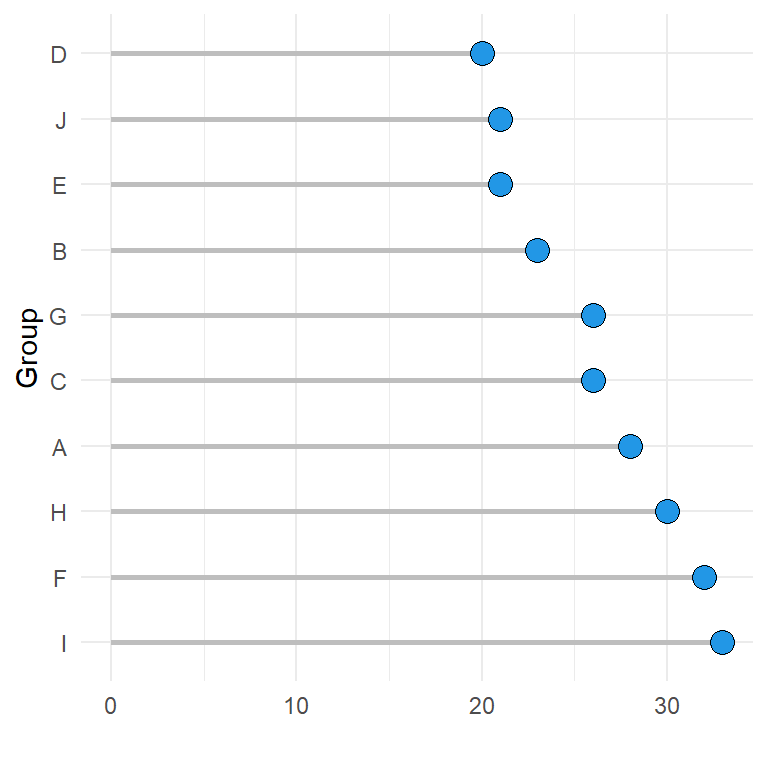# Lollipop chart in ggplot2

## Sample data

In this tutorial we are going to use the following data frame, which contains a numerical variable and a categorical variable representing each group.

``````# Sample data set
set.seed(1)
df <- data.frame(x = LETTERS[1:10],
y = sample(20:35, 10, replace = TRUE))``````

## Basic lollipop chart

Basic lollipop chart

Given a data frame with a categorical and a numerical variable it is possible to create a lollipop chart in ggplot2 combining `geom_segment` and `geom_point`.

``````# install.packages("ggplot2")
library(ggplot2)

ggplot(df, aes(x = x, y = y)) +
geom_segment(aes(x = x, xend = x, y = 0, yend = y)) +
geom_point() ``````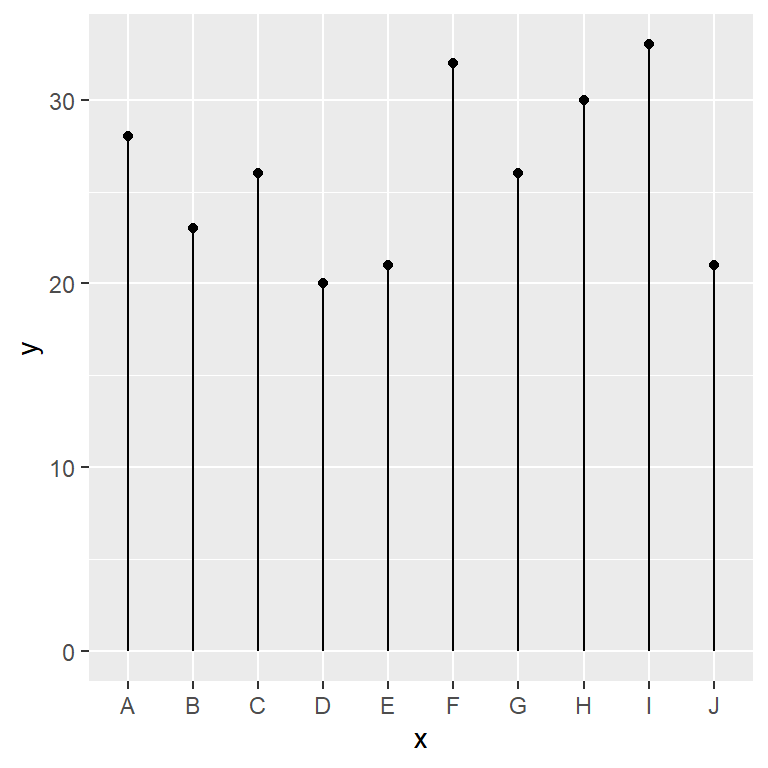Horizontal lollipop chart

Note that in several scenarios, specially if the labels of the categories are long it is recommended to create a horizontal chart. For that purpose you can use `coord_flip` or flip the order of the variables inside `aes`.

``````# install.packages("ggplot2")
library(ggplot2)

ggplot(df, aes(x = x, y = y)) +
geom_segment(aes(x = x, xend = x, y = 0, yend = y)) +
geom_point() +
coord_flip()``````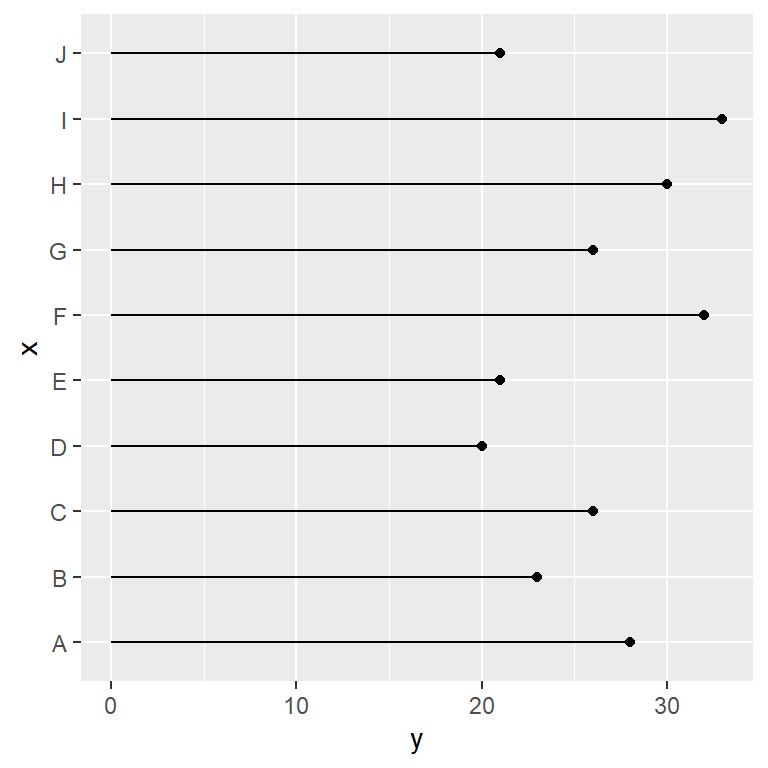## Lollipop customization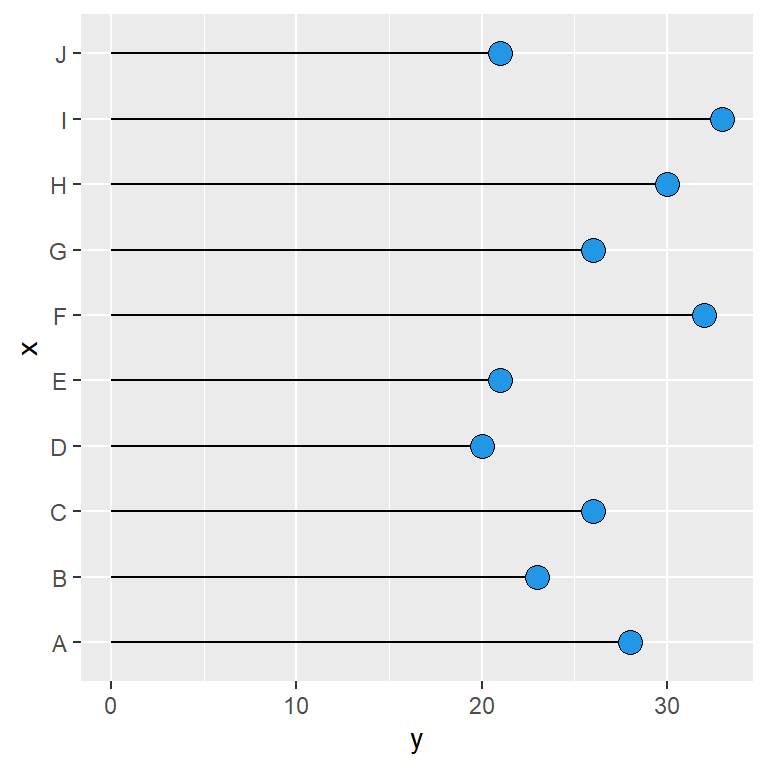Points

The points can be customized passing arguments to the `geom_point` function. In the following example we are changing the size, the symbol, the background color and the border of the symbol.

``````# install.packages("ggplot2")
library(ggplot2)

ggplot(df, aes(x = x, y = y)) +
geom_segment(aes(x = x, xend = x, y = 0, yend = y)) +
geom_point(size = 4, pch = 21, bg = 4, col = 1) +
coord_flip()``````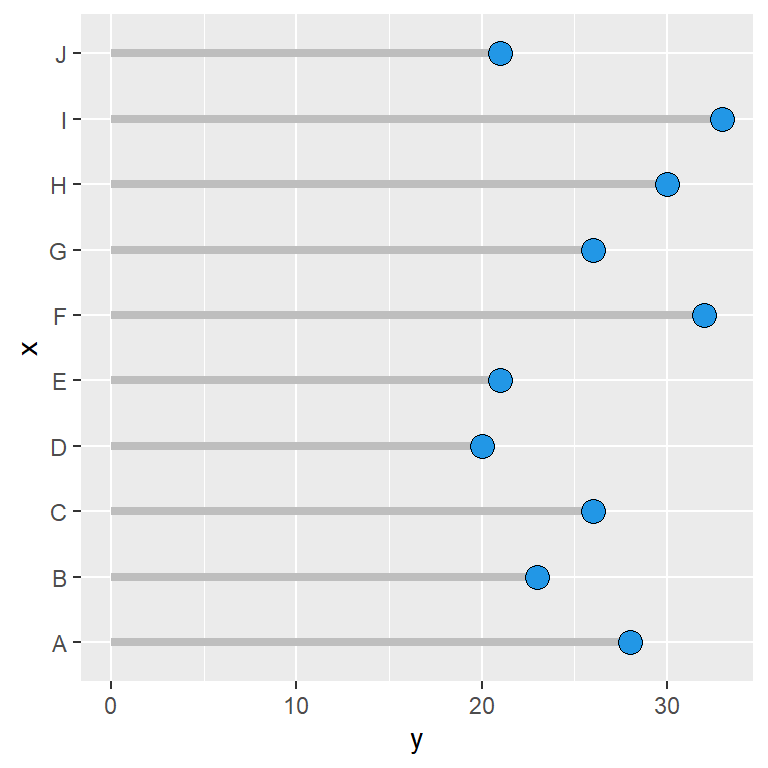Segments

Similarly to customizing the points you can also customize the segments. In the example below we are setting its color to gray and increasing its width.

``````# install.packages("ggplot2")
library(ggplot2)

ggplot(df, aes(x = x, y = y)) +
geom_segment(aes(x = x, xend = x, y = 0, yend = y),
color = "gray", lwd = 1.5) +
geom_point(size = 4, pch = 21, bg = 4, col = 1) +
coord_flip()``````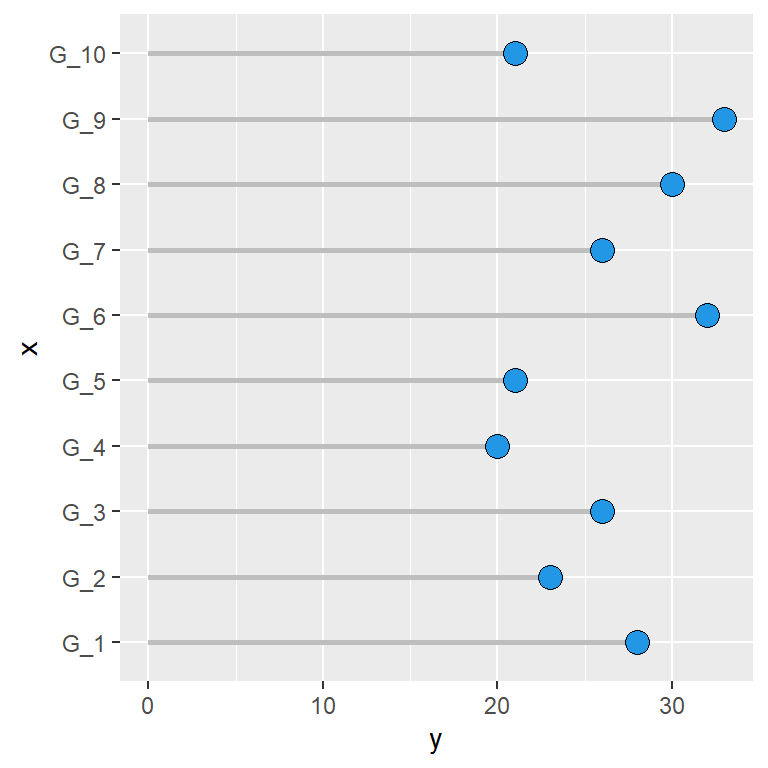Custom labels

As the X-axis is discrete it is possible to change the labels of the categories with the `labels` argument of `scale_x_discrete`.

``````# install.packages("ggplot2")

ggplot(df, aes(x = x, y = y)) +
geom_segment(aes(x = x, xend = x, y = 0, yend = y),
color = "gray", lwd = 1) +
geom_point(size = 4, pch = 21, bg = 4, col = 1) +
scale_x_discrete(labels = paste0("G_", 1:10)) +
coord_flip()``````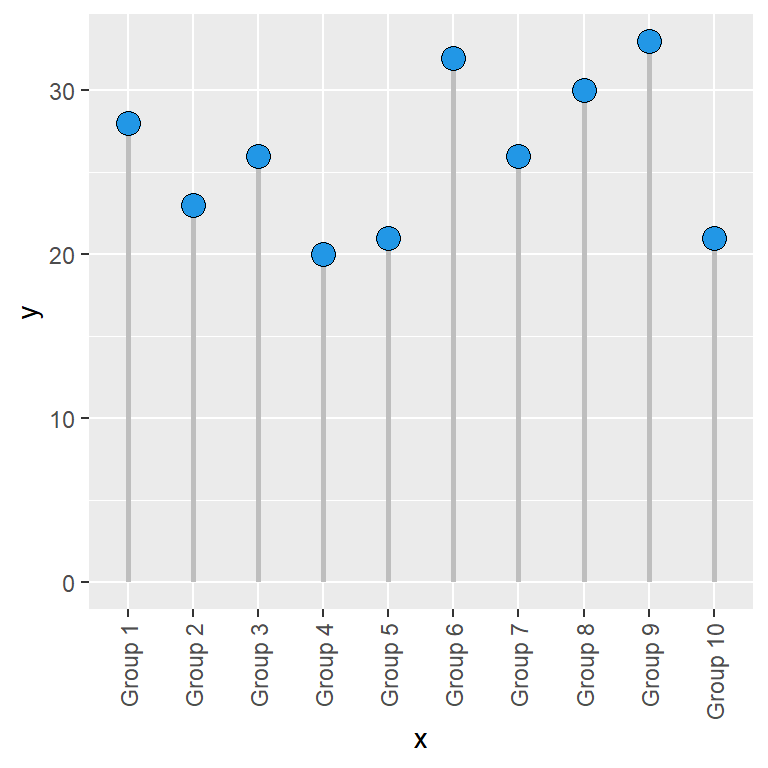Rotate the labels

If the labels are too long they won’t fit. However, you can rotate the labels with the `angle` argument of the `element_text` function for the `axis.text.x` theme component.

``````# install.packages("ggplot2")
library(ggplot2)

ggplot(df, aes(x = x, y = y)) +
geom_segment(aes(x = x, xend = x, y = 0, yend = y),
color = "gray", lwd = 1) +
geom_point(size = 4, pch = 21, bg = 4, col = 1) +
scale_x_discrete(labels = paste("Group", 1:10)) +
theme(axis.text.x = element_text(angle = 90,
vjust = 0.5, hjust = 1))``````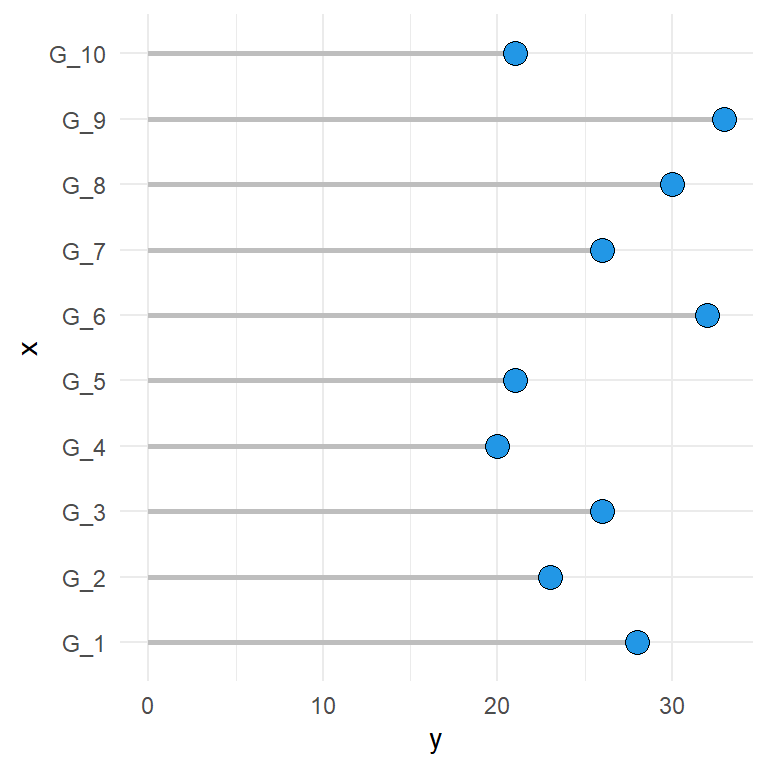Theme

As in other plots made with ggplot it is possible to change the theme of the plot.

``````# install.packages("ggplot2")
library(ggplot2)

ggplot(df, aes(x = x, y = y)) +
geom_segment(aes(x = x, xend = x, y = 0, yend = y),
color = "gray", lwd = 1) +
geom_point(size = 4, pch = 21, bg = 4, col = 1) +
scale_x_discrete(labels = paste0("G_", 1:10)) +
coord_flip() +
theme_minimal()``````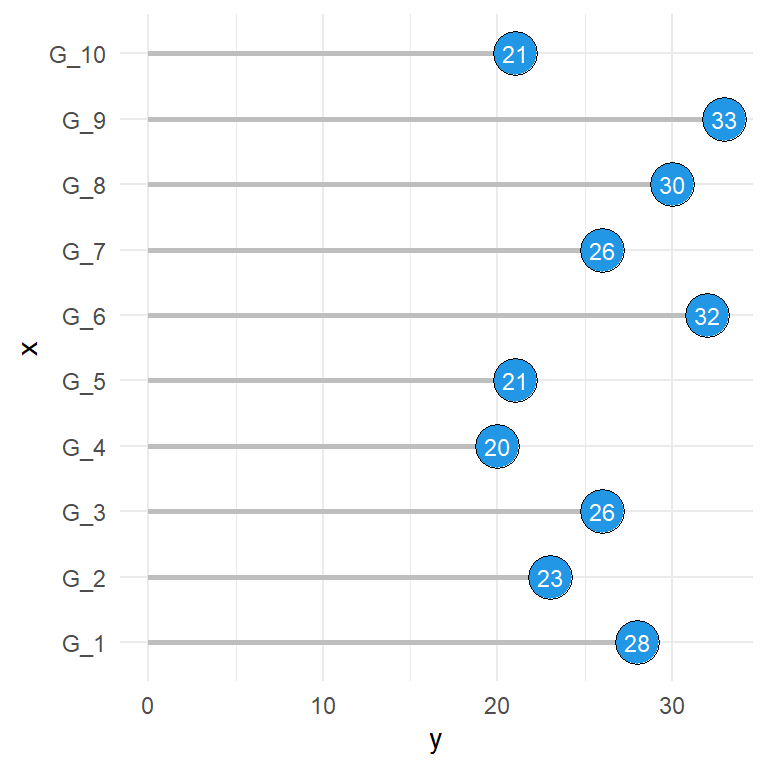Text

Note that you can also add the corresponding values or other text inside the points making use of `geom_text`.

``````# install.packages("ggplot2")
library(ggplot2)

ggplot(df, aes(x = x, y = y)) +
geom_segment(aes(x = x, xend = x, y = 0, yend = y),
color = "gray", lwd = 1) +
geom_point(size = 7.5, pch = 21, bg = 4, col = 1) +
geom_text(aes(label = y), color = "white", size = 3) +
scale_x_discrete(labels = paste0("G_", 1:10)) +
coord_flip() +
theme_minimal()``````

## Lollipop order

Finally, you can reorder the lollipops reordering the levels of the categorical variable or making use of `reorder` or an equivalent function.

``````# install.packages("ggplot2")
library(ggplot2)

ggplot(df, aes(x = reorder(x, -y), y = y)) +
geom_segment(aes(x = reorder(x, -y),
xend = reorder(x, -y),
y = 0, yend = y),
color = "gray", lwd = 1) +
geom_point(size = 4, pch = 21, bg = 4, col = 1) +
xlab("Group") +
ylab("") +
coord_flip() +
theme_minimal()``````